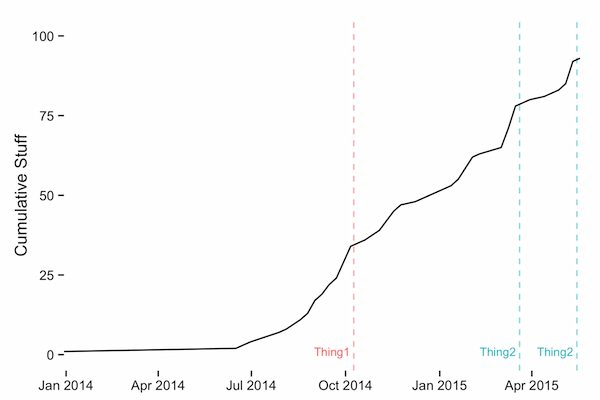Want to share your content on R-bloggers? click here if you have a blog, or here if you don't.

I set aside a small bit of time to give rbokeh a try and figured I’d share a small bit of code that shows how to make the “same” chart in both ggplot2 and rbokeh.

#### What is Bokeh/rbokeh?

rbokeh is an htmlwidget wrapper for the Bokeh visualization library that has become quite popular in Python circles. Bokeh makes creating interactive charts pretty simple and rbokeh lets you do it all with R syntax.

#### Comparing ggplot & rbokeh

This is not a comprehensive introduction into rbokeh. You can get that here (officially). I merely wanted to show how a ggplot idiom would map to an rbokeh one for those that may be looking to try out the rbokeh library and are familiar with ggplot. They share a very common “grammar of graphics” base where you have a plot structure and add layers and aesthetics. They each do this a tad bit differently, though, as you’ll see.

First, let’s plot a line graph with some markers in ggplot. The data I’m using is a small time series that we’ll use to plot a cumulative sum of via a line graph. It’s small enough to fit inline:

``` library(ggplot2) library(rbokeh) library(htmlwidgets)   structure(list(wk = structure(c(16069, 16237, 16244, 16251, 16279, 16286, 16300, 16307, 16314, 16321, 16328, 16335, 16342, 16349, 16356, 16363, 16377, 16384, 16391, 16398, 16412, 16419, 16426, 16440, 16447, 16454, 16468, 16475, 16496, 16503, 16510, 16517, 16524, 16538, 16552, 16559, 16566, 16573), class = "Date"), n = c(1L, 1L, 1L, 1L, 3L, 1L, 3L, 2L, 4L, 2L, 3L, 2L, 5L, 5L, 1L, 1L, 3L, 3L, 3L, 2L, 1L, 1L, 1L, 2L, 1L, 2L, 7L, 1L, 2L, 6L, 7L, 1L, 1L, 1L, 2L, 2L, 7L, 1L)), .Names = c("wk", "n"), class = c("tbl_df", "tbl", "data.frame"), row.names = c(NA, -38L)) -> by_week   events <- data.frame(when=as.Date(c("2014-10-09", "2015-03-20", "2015-05-15")), what=c("Thing1", "Thing2", "Thing2")) ```

The ggplot version is pretty straightforward:

``` gg <- ggplot() gg <- gg + geom_vline(data=events, aes(xintercept=as.numeric(when), color=what), linetype="dashed", alpha=1/2) gg <- gg + geom_text(data=events, aes(x=when, y=1, label=what, color=what), hjust=1.1, size=3) gg <- gg + geom_line(data=by_week, aes(x=wk, y=cumsum(n))) gg <- gg + scale_x_date(expand=c(0, 0)) gg <- gg + scale_y_continuous(limits=c(0, 100)) gg <- gg + labs(x=NULL, y="Cumulative Stuff") gg <- gg + theme_bw() gg <- gg + theme(panel.grid=element_blank()) gg <- gg + theme(panel.border=element_blank()) gg <- gg + theme(legend.position="none") gg ```

We:

• setup a base ggplot object
• add a layer of marker lines (which are the 3 `events` dates)
• add a layer of text for the marker lines
• add a layer of the actual line – note that we can use `cumsum(n)` vs pre-compute it
• setup scale and other aesthetic properties

That gives us this:Here’s a similar structure in rbokeh:

``` figure(width=550, height=375, logo="grey", outline_line_alpha=0) %>% ly_abline(v=events\$when, color=c("red", "blue", "blue"), type=2, alpha=1/4) %>% ly_text(x=events\$when, y=5, color=c("red", "blue", "blue"), text=events\$what, align="right", font_size="7pt") %>% ly_lines(x=wk, y=cumsum(n), data=by_week) %>% y_range(c(0, 100)) %>% x_axis(grid=FALSE, label=NULL, major_label_text_font_size="8pt", axis_line_alpha=0) %>% y_axis(grid=FALSE, label="Cumulative Stuff", minor_tick_line_alpha=0, axis_label_text_font_size="10pt", axis_line_alpha=0) -> rb rb ```

Here, we set the `width` and `height` and configure some of the initial aesthetic options. Note that `outline_line_alpha=0` is the equivalent of `theme(panel.border=element_blank())`.

The markers and text do not work exactly as one might expect since there’s no way to specify a `data` parameter, so we have to set the colors manually. Also, since the target is a browser, points are specified in the same way you would with CSS. However, it’s a pretty easy translation from `geom_[hv]line` to `ly_abline` and `geom_text` to `ly_text`.

The `ly_lines` works pretty much like `geom_line`.

Notice that both ggplot and rbokeh can grok dates for plotting (though we do not need the `as.numeric` hack for rbokeh).

rbokeh will auto-compute bounds like ggplot would but I wanted the scale to go from 0 to 100 in each plot. You can think of `y_range` as `ylim` in ggplot.

To configure the axes, you work directly with `x_axis` and `y_axis` parameters vs `theme` elements in ggplot. To turn off only lines, I set the alpha to 0 in each and did the same with the y axis minor tick marks.

Here’s the rbokeh result:

NOTE: you can save out the widget with:

``` saveWidget(rb, file="rbokeh001.html") ```

and I like to use the following `iframe` settings to include the widgets:

``` ```

#### Wrapping up

Hopefully this helped a bit with translating some ggplot idioms over to rbokeh and developing a working mental model of rbokeh plots. As I play with it a bit more I’ll add some more examples here in the event there are “tricks” that need to be exposed. You can find the code up on github and please feel free to drop a note in the comments if there are better ways of doing what I did or if you have other hints for folks.